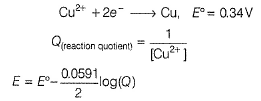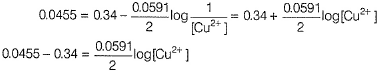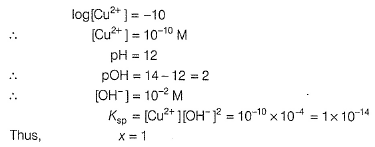Courses

# Test: Measurements: Applications of EMF

## 19 Questions MCQ Test Chemistry Class 12 | Test: Measurements: Applications of EMF

Description
This mock test of Test: Measurements: Applications of EMF for JEE helps you for every JEE entrance exam. This contains 19 Multiple Choice Questions for JEE Test: Measurements: Applications of EMF (mcq) to study with solutions a complete question bank. The solved questions answers in this Test: Measurements: Applications of EMF quiz give you a good mix of easy questions and tough questions. JEE students definitely take this Test: Measurements: Applications of EMF exercise for a better result in the exam. You can find other Test: Measurements: Applications of EMF extra questions, long questions & short questions for JEE on EduRev as well by searching above.
QUESTION: 1

### Only One Option Correct Type This section contains 11 multiple choice questions. Each question has four choices (a), (b), (c) and (d), out of which ONLY ONE is correct Q.  For, Pt(H2)/H2O, electrode potential at 298 K and 1 bar is

Solution:

It is an anode and thus oxidation takes place: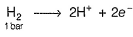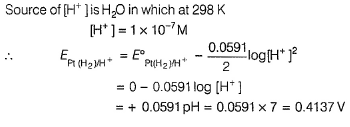QUESTION: 2

### For the cell reaction at 298K, Cu2+(aq) + 2e- → Cu(s) Variation of log[Cu2+ ] with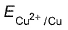is a straight line of intercept 0.34 V onaxis .Then electrode potential of the half-cell. Cu/Cu2+ (0.1 M) will be

Solution:

Cu2+(aq) + 2e- → Cu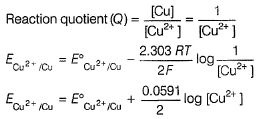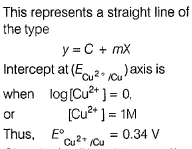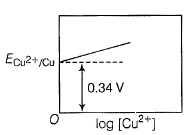Given half-cell is oxidation half-cell (anode)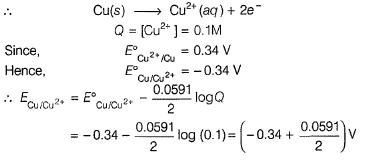QUESTION: 3

### Given, Cu2+ + 2e- → Cu, E° = + 0.34V, Ksp of Cu(OH)2 = 1.0 x 10-19 What i s E°red of Cu2+/Cu couple at pH = 12?

Solution:

pH = 12
pOH = 2, [OH-] = 10-2M

Cu(OH)2,(s)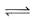cu2+ (aq) + 2OH(aq)
∴    Ksp = [Cu2+][OH-]2
1 x 10-19 = [Cu2+][102-]2

∴ Cu2+ + 2e→ cu

Reaction Quotient (Q) =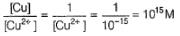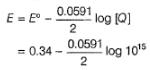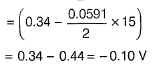QUESTION: 4

EMF of the following cell is 0.67 V at 298K.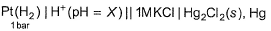Thus, pH of the solution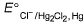= 0.28 V

Solution:

Hydrogen electrode has been coupled to calomel electrode.

Anode
H2(g) → 2H+(aq) + 2e     E0SHE = 0.00

Cathode
Hg2Cl2(s) + 2e- .→ 2 Hg(e)+2Cl- (aq)      E0cal, = 0.28 V

Hg2Cl2(s) + H2(g) → 2Hg(l) + 2H+(aq) + 2Cl- (aq)      E0cell, = 0.28 V

Reaction quotient (Q) =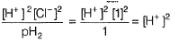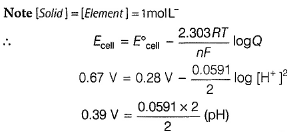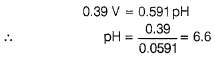QUESTION: 5

Given,

A3+ + e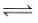A2+  , E0. = 1.42 V

B4+ + 2e-B2+   , E0. = 0.40 V

In the potentiometric titration of B2+ with A3+ the potential at the equivalence point is

Solution: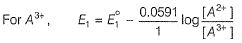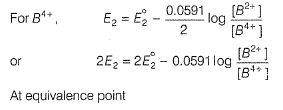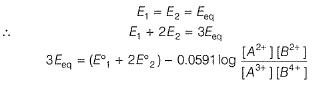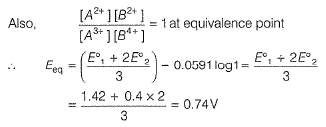QUESTION: 6

For the cell of 298 K,

Zn(s) + Cu2+ (aq)Cu(s) + Zn2+ (aq)

Variation of Ecell with logQ (where Q is reaction quotient ) is of the type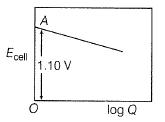At what value of ratio of molar  concentration of ions Ecell   would be 1.1591?

Solution: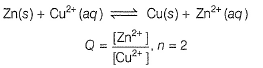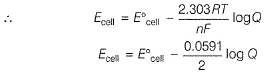This equation represents a straight line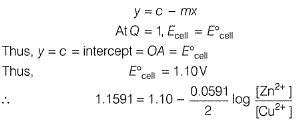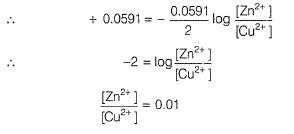QUESTION: 7

Given, Ag+ + e → Ag,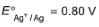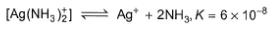Thus, E° of the following half-cell reaction at 298 K.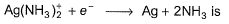Solution: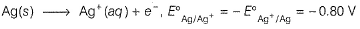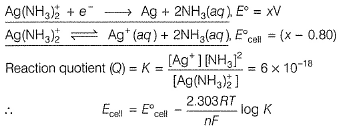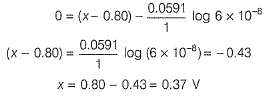QUESTION: 8

Ag/Ag electrode is immersed in 1.00 M KCl at 298 K. Ksp (AgCl) = 1.0 x 10-10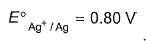Thus, EMF of the cell set up is

Solution:

In this case Ag+ ion collects as solid AgCI on the electrode itself. Some Ag+ exists in equilibrium with AgCI(s) in solution.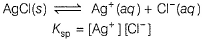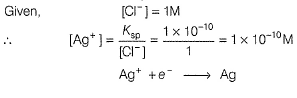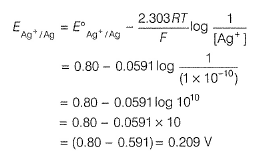QUESTION: 9

For the following cell, Pt(H2) | HCI(aq) || AgCI | Ag

Ecell = 0.2650 V at 298 K and Ecell = 0.2595 V at 308 K

Thus, heat of reaction at 298 K is

Solution: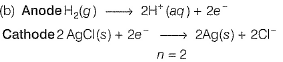By Gibbs Helmholtz equation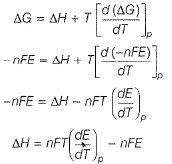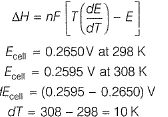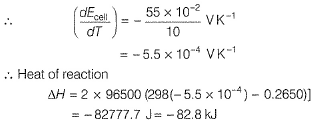QUESTION: 10

Quinhydrone electrode is an indicator electrode in contact with platinum metal and can be used in acid-base potentiometric titration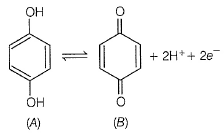At the equivalence point, [A] = [B] and thus, EMF of the cell is dependent on pH. When NaOH is added to HCl in potentiometric titration, potentiogram is

Solution: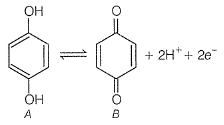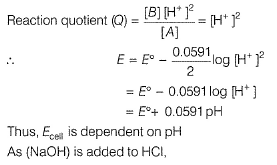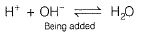Initially in acid (HCI), pH < 7 at neutral point pH = 7 and after HCI is neutralised, pH increases due to further addition of NaOH. Hence, Ecell also increases.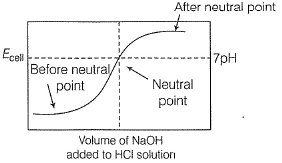QUESTION: 11

Potential at the equivalence point in the potentiometric titration of Fe2+ with MnO4- in acidic medium is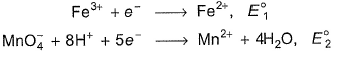Solution:

When equivalence point is reached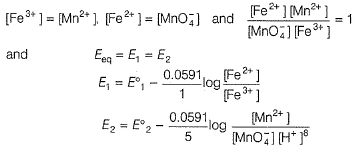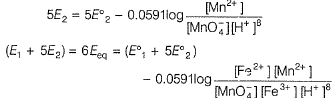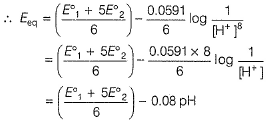QUESTION: 12

Comprehension Type

Direction : This section contains 3 paragraphs, each describing theory, experiments, data, etc. Six questions related to the paragraph have been given. Each question has only one correct answer among the four given options (a), (b), (c) and (d).

Passage I

The electrochemical cell shown below is a concentration cell :

M/Mz+ (saturated solution of a sparingly soluble salt(MX2) || M2+(0.001 mol dm-3)|M

The EMF of the cell depends on the difference in concentration of M2+ ions at the two electrodes .The EMF of the cell at 298 K is 0.059 V.

Q.

Ksp mol3 dm-9 (the solubility product) of MX2 at 298 K is

(2.303 RT/F = 0.059 V at 298 K)

Solution: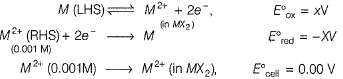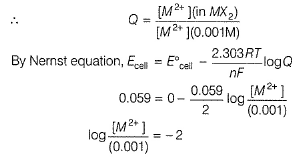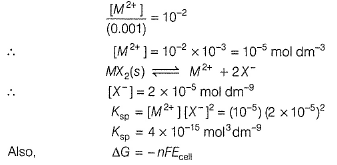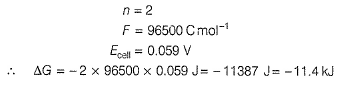QUESTION: 13

Passage I

The electrochemical cell shown below is a concentration cell :

M/Mz+ (saturated solution of a sparingly soluble salt(MX2) || M2+(0.001 mol dm-3)|M

The EMF of the cell depends on the difference in concentration of M2+ ions at the two electrodes .The EMF of the cell at 298 K is 0.059 V.

Q.

The value of ΔG (kJ mol-1) is (1F = 96500 C mol-1)

Solution:QUESTION: 14

Passage II

The standard half-cell reduction potential of

Fe3+(aq) | Fe is -0.036V and that of OH- | Fe(OH3)(s) | Feis -0.786V.

Q.

For the determination of solubility product of Fe(OH)3 (Ksp) the appropriate cell representation and its EMF respectively are

Solution: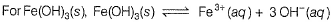Above reaction is the net reaction in a cell for determination of Ksp.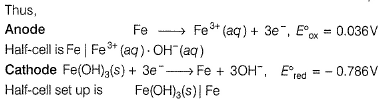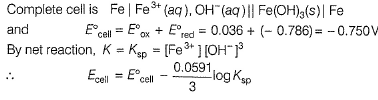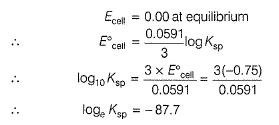QUESTION: 15

Passage II

The standard half-cell reduction potential of

Fe3+(aq) | Fe is -0.036V and that of OH- | Fe(OH3)(s) | Feis -0.786V.

Q.

The value of loge Ksp for Fe(OH)3 at 298 K is

Solution:Above reaction is the net reaction in a cell for determination of Ksp.

Thus,

Anode

Fe → Fe3+ (aq) + 3e-, E0ox = 0.036V

Half - cell is Fe|Fe3+ (aq). OH-(aq)

Cathode

Fe(OH)3(s) + 3e→ Fe+ 3OH-, E0red = -0.786 V

Half-cell set up is Fe(OH)3(s)|Fe

complete cell is Fe|Fe3+(aq), OH- (aq)||Fe(OH)3 (s)|Fe

and E0cell = E0ox + E0red = 0.036 + (-0.786) = -0.750V

By net reaction, K = Ksp = [Fe3+][OH-]3

∴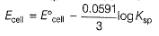QUESTION: 16

Passage III

Tollen's reagent is used for the detection of aldehydes.When a solution of AgNOis added to glucose with NH4OH, then gluconic acid is formed.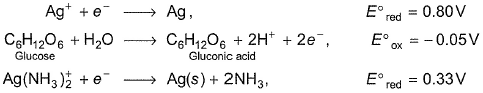Q.

For the reaction,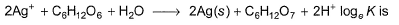Solution:

2Ag+ + 2e- → 2Ag

C6H12O6 +H2O → C6H12O7 + 2H+ + 2e-
E0r = 0.80V  E0 = -0.05V

2Ag+ + C6H12O6 + H2O → 2Ag+ C6H12O+ 2H+

E0cell = 0.75 V

At equilibrium,  Ecell = 0When NH3 is added, pH increases to 11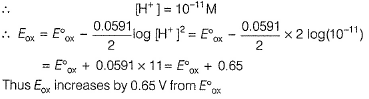QUESTION: 17

Passage III

Tollen's reagent is used for the detection of aldehydes.When a solution of AgNOis added to glucose with NH4OH, then gluconic acid is formed.Q.

In the presence of NH3, pH is raised to 11.Thus,

Solution: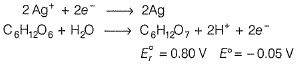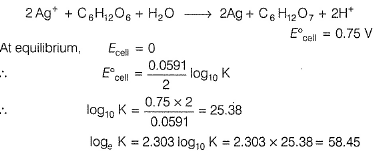When NH3 is added, pH increases to 11*Answer can only contain numeric values
QUESTION: 18

One Integer Value Correct Type

This section contains 2 questions, when worked out will result in an integer value from 0 to 9 (both inclusive)

Q.

For the following half-cell,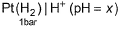EMF at 298 K is 0.2364 V. What is pH?

Solution: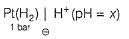It is oxidation half-cell, and anode reaction is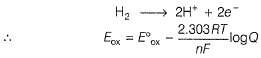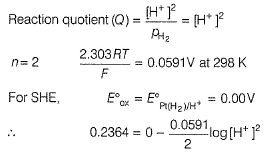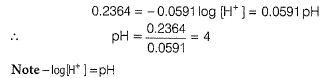*Answer can only contain numeric values
QUESTION: 19

For Cu(OH)2|Cu hall-cell at pH = 12, electrode potential is 0.0455 at 298 K.

For Cu2+ (aq) + 2e- → Cu, E° = 0.34 V. Ksp value of Cu(OH)2 is x x 10-4

What is the value of x?

Solution: